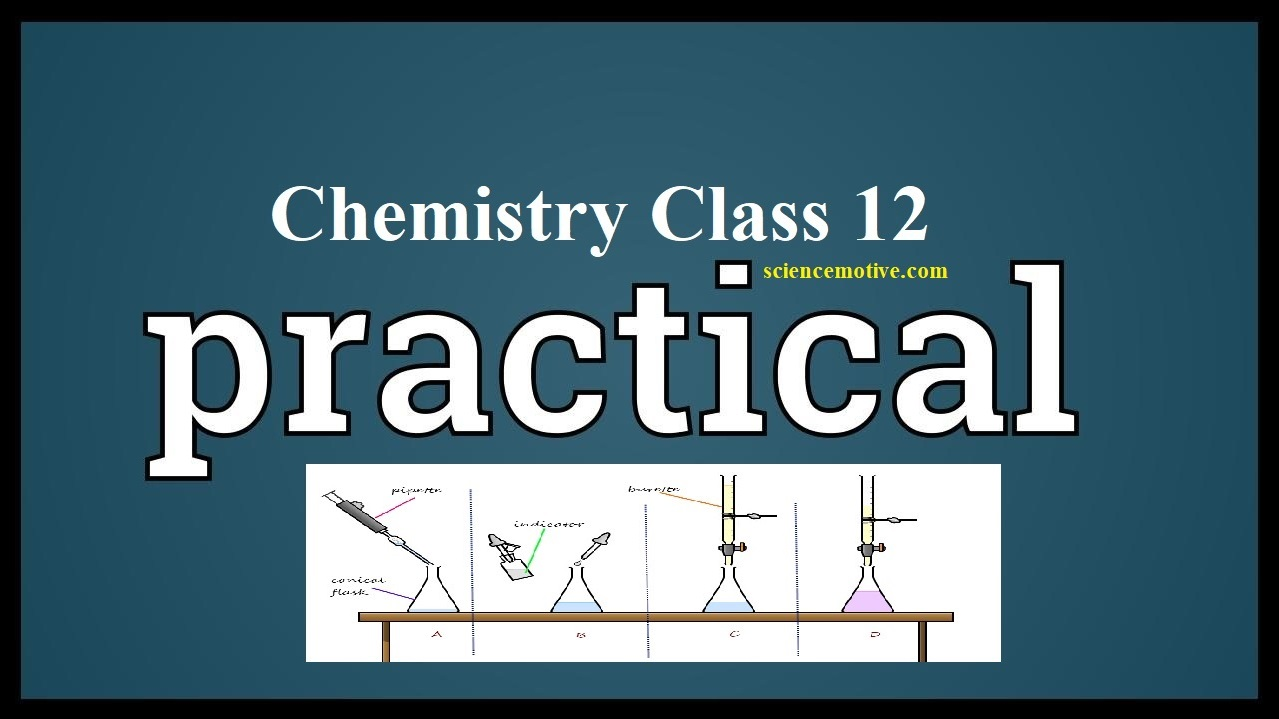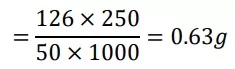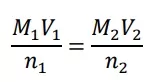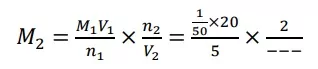# Chemistry Practical Class 12# Chemistry Practical Class 12

Chemistry Practical Class 12

## TITRATION or VOLUMETRIC ANALYSIS

Aim: – To prepare M/50 Oxalic acid solution and determine the molarity, strength of the given potassium permanganate solution.

Theory: In this titration oxalic acid acts as a reducing agent and potassium permanganate acts as an oxidising agent. So, the reaction between oxalic acid and potassium permanganate is a redox reaction. In this redox reaction, carbon of oxalic acid gets oxidized to carbon dioxide and manganese present in potassium permanganate, which is in the +7 oxidation state gets reduced to colourless Mn2+ state.

Chemistry Practical Class 12

Chemical equation:
Ionic Half equations
𝑀nO4 + 8𝐻+ + 5𝑒 → 𝑀n2+ + 4H2O] × 2
C2O42 → 2𝐶O2 + 2𝑒    ] × 5

2𝑀𝑛O4 + 16𝐻+ + 5C2O42− → 2 𝑀𝑛2+ + 8𝐻2O + 10CO2    (Over all Ionic equation)
Molecular Half Equations →
2𝐾𝑀𝑛O4 + 3H2𝑆O4 → K2𝑆O4 + 2𝑀𝑛𝑆O4 + 3H2𝑂 + 5[𝑂]
{(COOH)2. 2H2O + [O] → 2CO2 + 3H2O}×5

2𝐾𝑀𝑛O4 + (COOH)2. 2H2O + 3H2𝑆O4 → K2𝑆O4 + 2𝑀𝑛𝑆O4 + 10CO2 + 18H2O (Over all Molecular equation).

Chemistry Practical Class 12

Chemicals Required:
1. Oxalic acid, Potassium permanganate solution
2. Dilute sulphuric acid
3. Distilled water

Apparatus Required:
1. Electronic balance
2. Beakers
3. Burette stand
4. Burette
5. Pipette
7. Spatula
8. Funnel

Indicator: – KMnO4 act as a self-indicator

Endpoint:- Colourless to permanent pink colour [KMnO4 in burette]

Chemistry Practical Class 12

(i) Preparation of M/50 oxalic acid solution:
The molar mass of oxalic acid (COOH)2. 2H2O) is 126 g/mol. For the preparation of
250 mL of M/50 oxalic acid solution, the amount of oxalic acid required isso, dissolve 0.63g of oxalic acid into 250 ml of water. Now the solution is M/50 oxalic acid of volume 250 mL.

(ii) Titration of potassium permanganate solution against standard oxalic acid
solution:
1. Wash the burette, pipette, conical flask and funnel with distilled water.
2. Rinse the burette with the potassium permanganate solution and fill the burette with potassium permanganate solution.
3. Fix the burette in the burette stand and place the white tile below the burette in
order to find the endpoint correctly.
4. Rinse the pipette with the standard oxalic acid solution.
5. Pipette out 20 mL of standard oxalic acid solution into the conical flask.
6. Add a test tube full of dilute sulphuric acid to the conical flask
7. Heat the mixture up to 50 𝑡𝑜 600C before titrating it with potassium
permanganate.
8. Note down the initial reading in the burette before starting the titration.
9. Add potassium permanganate solution from burette to the solution in the flask
with stirring.
10. Initially, the pink colour of KMnO4 is discharged with the oxalic acid solution.
The appearance of a permanent pink colour indicates the endpoint.
11. At the endpoint, note down the upper meniscus on the burette readings.
12. Repeat the titration until concordant values are obtained.
13. Record the reading in the observation table.

Chemistry Practical Class 12

Observation Table:

 S.No. Initial Reading (IR) in ml Final Reading (FR) in ml The volume of KmnO4 Used (FR-IR) in ml 1 2 3

Concordant Volume of KMnO4 used = _____ mL

Calculations:
(a) Molarity calculation:- Using Molarity EquationM1 = Molarity of Oxalic acid solution=1/50
M2 = Molarity of KMnO4 =?
V1 = Volume of Oxalic acid solution= 20 mL
V2 = Volume of KMnO4 used = Concordant reading i.e ______ mL
According to the overall ionic equation 2 moles of 𝑀𝑛𝑂4 reacts with 5 moles of oxalate ion (C2O42)
n1 = 5, 𝑎𝑛𝑑 n2 = 2(b) Strength of KMnO4 solution = Molarity × Molar mass of KMnO4   = ______ × 158 g/L     =________ g/L

Result: Molarity = _____ M
Strength = _____ g/L

Precautions:
1. Do not blow the air from the mouth or jerk the pipette while removing the last drop of solution. Only touch it with the bottom.
2. Rinse the burette and pipette with the solution to be used in them.
3. Do not rinse the titration flask.
4. Remove the air bubble from the nozzle of the burette
5. Read the upper meniscus for coloured solution

Chemistry Practical Class 12